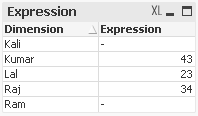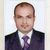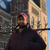Announcements
Sept. 27, 10AM ET Do more with Qlik, Insight Advisor – our intelligent AI-assistant in Qlik Sense: Register
cancel
Showing results for
Did you mean:Partner - Contributor III

## Count straight table rows

Hi All,

Kindly help me to count the number of rows in a straight table.

Example:Note : '-' is Null in the above screenshot.

I have tried count(distinct Dimension) , I am getting 5.But the expected count is 3 excluding Nulls.

Thanks

Dinesh M

9 RepliesMVP

Try expression

count(distinct If(Dimension <> '-' or Dimension = isnull() or Dimension = 0,  Dimension) )MVP

try below expression

=count(distinct {<Dimension={"=(Expression)>0"}>}Dimension)MVP

Another version

=Count( Distinct {<Dimension -= {"=(Isnull(Dimension)) = -1"}>} Dimension )Anonymous
Not applicable

Hi

Try this  SetAnalysis

=count(distinct {<Dimension={"=(Expression)>0"}>}Dimension)

It will Suppress your Null value OR Value with 0

Regards

Tahemas MominChampion

Hi Dinesh,

Try this on the script :

if(expression <> '' or not isnull(expression),1,0) as count

Regards,

YoussefMVP

Try some of this

=Count(distinct If(Expression <> '-' or Expression = Isnull() or Expression = 0,  Dimension) )

Or

=Count(distinct If( Isnull(Expression) <> -1,  Dimension) )

Or

=Count( Distinct {<Expression-= {"=(Isnull(Expression)) = -1"}>} Dimension )MVPEmployee

Hello,

what about the following set analysis?

Count( DISTINCT {\$<Dimension = {"=NullCount(Expression) > 0"}>} Dimension)

Regards,

BriceMVP

I believe Expression is that you have some Metric calculation, if it is same than replace the Expression place with your expression.

Kindly check this also will it work for you.Community Browser